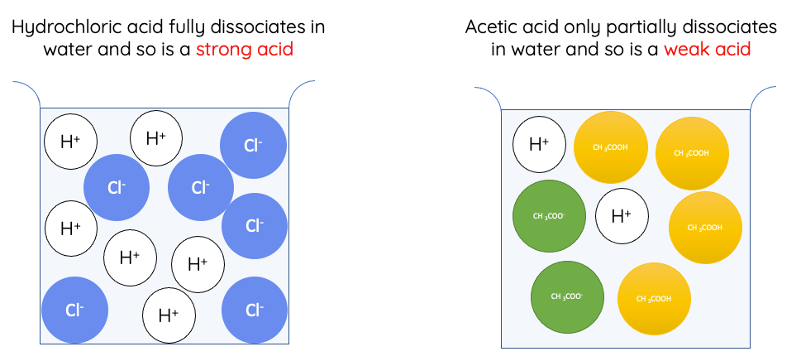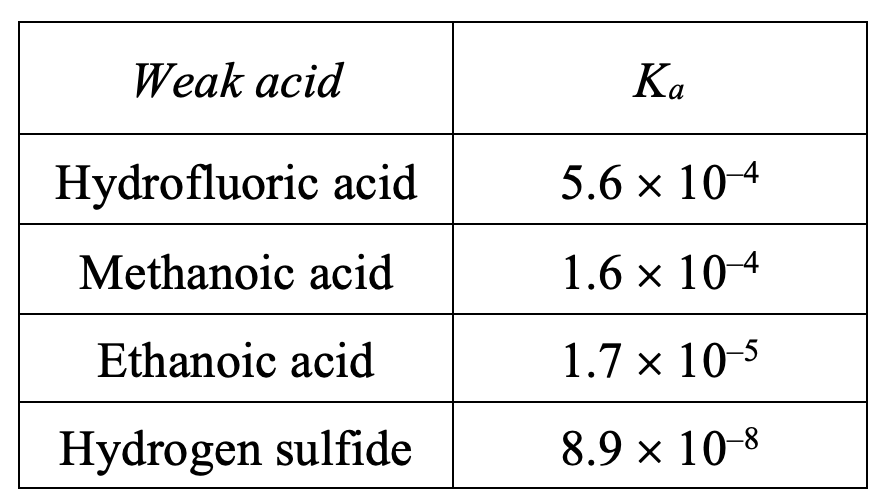# Acid and Base Dissociation Constants (Ka and Kb)

This is part of the HSC Chemistry course under the topic Dissociation of Acids and Bases

### HSC Chemistry Syllabus

• Explore the use of Keq for different types of chemical reactions, including but not limited to:

– dissociation of ionic solutions

– dissociation of acids and bases

### What are acid and base dissociation constants?

This video will explore acid and base dissociation constants, what they are, and how they are calculated.

### Introduction to Acids

• Acids, by various definitions, are hydrogen ion (H+) producers (Arrhenius theory) or proton donors (Brønsted-Lowry theory).
• Not all hydrogen–containing molecules are acids. Molecules are only considered acids if the hydrogen atoms can be ionised or given away. Hydrogen atoms that can be ionised are described as acidic.
• For example, hydrogen atoms in methane, CH4, are not easily ionisable therefore methane is not considered an acid.

Acid Dissociation/Ionisation

• Dissociation is a chemical process whereby a compound splits into ions. Ionic compounds (e.g. NaCl), acids and bases can dissociate in water.• Weak acids are ones which do not ionise fully when dissolved in water. In other words, molecules of weak acids only partially produce hydrogen ions or donate protons. Therefore, weak acids exist in dynamic equilibria when in solution.

• Organic acids (acids that contain carbon) are weak acids. Examples of this include methanoic acid (CH3OH), acetic acid (CH3COOH) and citric acid (C6H8O7).• Some inorganic acids (acids that do not contain carbon) are weak acids. An example of this is hydrofluoric acid (HF).

• Ethanoic acid (acetic acid, found in vinegar) is a typical weak acid which reacts with water to produce hydronium ions (H3O+) and ethanoate ions (CH3COO)

$$CH_3COOH(aq)+H_2O(l) \rightleftharpoons CH_3COO^–(aq) + H_3O^+(aq)$$

Under standard conditions, only about 1% of ethanoic acid molecules are ionised and converted into ethanoate ions. The rest 99% remain as simple ethanoic acid molecules.

### Acid Dissociation Constant (Ka)

• The strength of weak acids can be measured and quantified by the equilibrium constant of their dissociation reaction. For a generic weak acid, HA, dissolved in water:

$$HA(aq) + H_2O(l) \rightleftharpoons A^–(aq) + H_3O^+(aq)$$

The equilibrium constant or acid dissociation constant of this reaction is:

$$K_{eq} = \frac{[A^–][H_3O^+]}{[HA]}$$

Since the concentration of water is large and remains relatively constant compared to a weak acid,   can be removed from the expression.

• The dissociation constant for ethanoic acid is:
$$CH_3COOH(aq)+H_2O(l) \rightleftharpoons CH_3COO^–(aq) + H_3O^+(aq)$$
$$K_{eq} = \frac{[CH_3COO^–][H_3O^+]}{[CH_3COOH]}$$

• Acid strength is proportional to the value of Ka. Higher the value indicates higher concentration of hydrogen ions and more acids ionised. This corresponds to a stronger acid.

The table shows Ka values of some simple weak acids, listed in decreasing strength.### Calculations for Acid Dissociation Constant Ka

• For a generic weak acid HA:

$$HA(aq) + H_2O(l) \rightleftharpoons A^–(aq) + H_3O^+(aq)$$

• When acid molecules dissociate, the concentration of hydrogen ions, [H3O+] equals [A] at equilibrium.

Commons assumptions

1. When the dissociation constant, Ka, is small, the proportion of acid molecules that undergoes ionisation is small. As such, it can be assumed that the initial concentration of acid, HA, approximately equals its concentration at equilibrium. In other words, the change in [HA] during ionisation is negligible.

1. It can be assumed, during calculation, that self-ionisation of water does not affect [H3O+] of the solution and therefore, it does not contribute to the pH.

1. If the acid is diprotic or triprotic, it can be usually assumed that the second and third ionisation occur to a smaller extent than the first ionisation.

In other words, if Ka of first ionisation is already small, Ka of subsequent ionisation reactions can be disregarded. For example, hydrogen sulfide is diprotic and its two ionisation reactions are as follows:

H2S(aq) + H2O(l) ⇌ HS(aq) + H3O+(aq)        Ka1 = 8.9 × 10–8

HS(aq) + H2O(l) ⇌ S2–(aq) + H3O+(aq)           Ka2 = 1.3 × 10–14

This assumption does not apply to all weak acids and certainly not strong acids. Some weak acids ionise to a considerable extent, to which its initial concentration differs substantially with that at equilibrium.

Example 1 – Calculating [H+from Ka

The Ka of hypochlorous acid, HOCl, is 3.5 × 10–8.

A solution of hypochlorous acid, HOCl, is made by adding 0.0400 moles of HOCl to 500.0 mL of distilled water. What is the hydrogen ion concentration of the solution?

Step 1: Write a balanced equation and an expression for Ka

$$HOCl(aq) \rightleftharpoons H^+(aq) + OCl^–(aq)$$

$$K_a = \frac{[H^+][OCl^–]}{[HOCl]}$$

Step 2: Set-up an ICE table

 [HOCl] [H+] [OCl–] Initial $$\frac{0.0400}{0.5000} = 0.0800 mol L^{–1}$$ 0 0 Change –x +x +x Equilibrium 0.0800 –x x x

Step 3: Substitute equilibrium concentration expressions (in terms of x) into Ka expression:

$$3.5 \times 10^{–8} = \frac{[x][x]}{[0.0800–x]}$$

Step 4: By making the assumption: x is small as Ka is small, simplify the equation to find x:

$$3.5 \times 10^{–8} = \frac{x^2}{0.0800}$$

$$x = [H^+] = \sqrt{0.0800 \times 3.5 \times 10^{-8}}$$

$$[H^+] = 5.3 \times 10^{–5} mol L^{–1}$$

Example 2 – Calculating Ka from [H+]

A 0.750 mol L–1 solution of a particular weak acid, HA, has a [H+] = 3.4 xx 10^(–3) mol L–1 at 25ºC. Calculate its Ka.

Step 1: Write a balanced equation and an expression for Ka

$$HA(aq) + H_2O(l) \rightleftharpoons A^–(aq) + H_3O^+(aq)$$

$$K_a = \frac{[A^–][H_3O^+]}{[HA]}$$

Step 2: Calculate Ka from equilibrium expression.

$$K_a = \frac{[3.4 \times 10^{–3}][3.4 \times 10^{–3}]}{[0.750 – 3.4 \times 10^{–3}]}$$

$$K_a = 1.5 \times 10^{–5}$$

### Calculations for Base Dissociation Constant, Kb

• A base by definition, is a substance that gains hydrogen ions or protons in solution.

• The equilibrium constant for the equilibrium established when a weak base reacts with water is referred to as the base dissociation constant. For example, for a generic base B.

$$B(aq) + H_2O(l) \rightleftharpoons HB^+(aq) + OH^–(aq)$$

The base dissociation constant is:

$$K_b = \frac{[OH^–][HB^+]}{[B]}$$

• The base dissociation constant of carbonate ion in water:

$$CO_3^{2–}(aq) + H_2O(l) \rightleftharpoons HCO_3^–(aq) + OH^–(aq)$$

$$K_b = \frac{[OH^–][HCO_3^–]}{[CO_3^{2–}]}$$

Previous Section: Common Solubility Equilibrium Questions